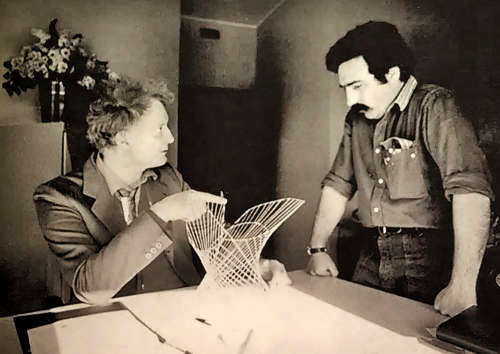### Regge theory

http://t.co/alaasqcHwl @ulaulaman says #goodbye to #TullioRegge
In quantum physics, Regge theory is the study of the analytic properties of scattering as a function of angular momentum, where the angular momentum is not restricted to be an integer but is allowed to take any complex value. The nonrelativistic theory was developed by Tullio Regge in 1957.
Following Chew and Frautschi (pdf), the key papers by Tullio Regge are:
Regge T. (1959). Introduction to complex orbital momenta, Il Nuovo Cimento, 14 (5) 951-976. DOI: http://dx.doi.org/10.1007/bf02728177 (pdf)
In this paper the orbital momentumj, until now considered as an integer discrete parameter in the radial Schrödinger wave equations, is allowed to take complex values. The purpose of such an enlargement is not purely academic but opens new possibilities in discussing the connection between potentials and scattering amplitudes. In particular it is shown that under reasonable assumptions, fulfilled by most field theoretical potentials, the scattering amplitude at some fixed energy determines the potential uniquely, when it exists. Moreover for special classes of potentials $V(x)$, which are analytically continuable into a function $V(z)$, $z=x+iy$, regular and suitable bounded in $x > 0$, the scattering amplitude has the remarcable property of being continuable for arbitrary negative and large cosine of the scattering angle and therefore for arbitrary large real and positive transmitted momentum. The range of validity of the dispersion relations is therefore much enlarged.
Regge T. (1960). Bound states, shadow states and mandelstam representation, Il Nuovo Cimento, 18 (5) 947-956. DOI: http://dx.doi.org/10.1007/bf02733035
In a previous paper a technique involving complex angular momenta was used in order to prove the Mandelstam representation for potential scattering. One of the results was that the number of subtractions in the transmitted momentum depends critically on the location of the poles (shadow states) of the scattering matrix as a function of the complex orbital momentum. In this paper the study of the position of the shadow states is carried out in much greater detail. We give also related inequalities concerning bound states and resonances. The physical interpretation of the shadow states is then discussed.
The importance of the model is summarized by the following:
As a fundamental theory of strong interactions at high energies, Regge theory enjoyed a period of interest in the 1960s, but it was largely succeeded by quantum chromodynamics. As a phenomenological theory, it is still an indispensable tool for understanding near-beam line scattering and scattering at very large energies. Modern research focuses both on the connection to perturbation theory and to string theory.
During the 1980s, Regge is interested also in the mathematical art, using Anschauliche Geometrie by David Hilbert and Stefan Cohn-Vossen like inspiration for a lot of mathematical objects.Good bye, Mr. Regge, and thanks for all...